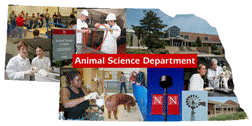## Animal Science Department## Faculty Papers and Publications in Animal Science

#### Date of this Version

12-19-1988

Published in Joural of Dairy Science Vol. 72, No, 6, 1989. Copyright 1989. Used by permission.

#### Abstract

Simultaneous genetic evaluations for bulls and cows obtained by mixed model procedures with an animal model incorporating all numerator relationships for artificially sired Holstein cows in northeastern United States were compared with separate (Northeast) mixed model evaluations of cows and sires for predicting genetic evaluations of progeny from dam, sire, and maternal grandsire evaluations. Regression coefficients for progeny (322,104 daughters and 837 sons) evaluations on dam, sire, and maternal grandsire evaluations were for daughters: Northeast evaluations; .50, .37, and -.02 compared to theoretical coefficients of .74, .52, and -.13, and simultaneous evaluations; .61, .50, and -.09 compared to theoretical coefficients of .73, .52, and -.12. For sons, comparable regression coefficients were: Northeast evaluations; .08, .42, and .06 compared to theoretical coefficients of .33, .59, and .00 and simultaneous evaluations; .92, .46 and -.29 compared to theoretical coefficients of 1.11, .54, and -.31. Efficiencies of evaluation procedures expressed as ratios of actual to theoretical multiple correlation coefficients were for daughters: Northeast evaluations, .70/.93 = .75; and simultaneous evaluations, .88/.93 = .95; and were for sons: Northeast evaluations, .50/.64 = .78; and simultaneous evaluations, .85/.89 = .95. Northeast evaluations were 79 and 82% as efficient as simultaneous evaluations for daughters and sons, respectively.

COinS